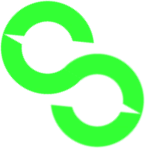statchute.xyz

 #60Subscriber Rank #66View Rank #55Video Rank 1797 +37.6%Subs in the last 30 days 607891 +34%Views in the last 30 days 48 +9.1%Videos in the last 30 days
DateSubs∆SubsViews∆ViewsVideos∆Videos
2020-10-24685701008613030830
2020-10-256885+281010963+235030830
2020-10-266962+771014342+33793084+1
2020-10-277004+421016271+19293086+2
2020-10-287072+681019547+32763088+2
2020-10-297138+661023202+36553091+3
2020-10-307192+541026786+35843095+4
2020-10-317235+431029679+28933096+1
2020-11-017277+421033433+37543099+3
2020-11-027331+541036730+329730990
2020-11-037356+251039613+28833101+2
2020-11-047378+221042451+28383103+2
2020-11-057433+551045748+32973108+5
2020-11-067508+751051874+61263114+6
2020-11-077560+521056628+47543116+2
2020-11-087599+391059473+28453117+1
2020-11-097645+461063450+39773118+1
2020-11-107702+571066587+31373121+3
2020-11-117767+651069878+32913123+2
2020-11-127851+841078627+87493127+4
2020-11-137905+541083876+52493129+2
2020-11-147989+841093928+100523134+5
2020-11-158112+1231104247+103193135+1
2020-11-168253+1411114603+103563136+1
2020-11-178328+751123115+85123141+5
2020-11-188398+701127811+46963143+2
2020-11-198515+1171135393+75823145+2
2020-11-208593+781141256+58633147+2
2020-11-218710+1171150243+89873149+2
2020-11-228920+2101159639+93963150+1
2020-11-239185+2651176594+169553152+2
2020-11-249225+401179570+29763153+1
2020-11-259345+1201186617+70473156+3
2020-11-269423+781193572+69553158+2
2020-11-279525+1021201885+83133161+3
2020-11-289706+1811213104+112193163+2
2020-11-299866+1601222510+94063165+2
2020-11-3010153+2871238184+156743166+1
2020-12-0110381+2281252155+139713168+2
2020-12-0210528+1471263414+112593170+2
2020-12-0310613+851272277+88633172+2
2020-12-0410690+771281223+89463174+2
2020-12-0510787+971290327+91043176+2
2020-12-0610878+911299156+88293177+1
2020-12-0711015+1371308098+89423178+1
2020-12-0811136+1211321282+131843181+3
2020-12-0911269+1331333770+124883183+2
2020-12-1011385+1161343681+99113185+2
2020-12-1111487+1021354064+103833187+2
2020-12-1211622+1351362480+84163188+1
2020-12-1312022+4001385728+232483190+2
2020-12-1412404+3821407162+214343185-5
2020-12-1512648+2441422053+148913186+1
2020-12-1612840+1921437509+154563188+2
2020-12-1713064+2241454795+172863190+2
2020-12-1813243+1791473840+190453194+4
2020-12-1913338+951489512+156723196+2
2020-12-2013462+1241503641+141293197+1
2020-12-2113692+2301521905+182643198+1
2020-12-2213795+1031533936+120313200+2
2020-12-2313836+411543625+96893202+2
2020-12-2413933+971556614+129893204+2
2020-12-2514128+1951577363+207493205+1
2020-12-2614301+1731594324+169613206+1
2020-12-2714586+2851616532+222083207+1
2020-12-2814763+1771639088+225563208+1
2020-12-2914931+1681661582+224943210+2
2020-12-3015083+1521679430+178483212+2
2020-12-3115190+1071698509+190793213+1
2021-01-0115328+1381721783+232743214+1
2021-01-0215454+1261738810+170273215+1
2021-01-0315554+1001752374+135643216+1
2021-01-0415721+1671769851+174773217+1
2021-01-0515799+781780968+111173218+1
2021-01-0615903+1041795934+149663220+2
2021-01-0715949+461803174+72403222+2
2021-01-0816069+1201819135+159613224+2
2021-01-0916243+1741835830+166953226+2
2021-01-1016866+6231893302+574723228+2
2021-01-1117532+6661973282+799803231+3
2021-01-1217921+3892013080+397983233+2
2021-01-1318196+2752037043+2396332330
2021-01-1418340+1442051529+144863235+2
2021-01-1518729+3892075678+241493236+1
2021-01-1619282+5532106114+304363238+2
2021-01-1719876+5942152991+468773239+1
2021-01-1820192+3162174880+2188932390
2021-01-1920456+2642206559+316793243+4
2021-01-2021097+6412272924+663653246+3
2021-01-2121401+3042311254+383303248+2
2021-01-2221580+1792336570+253163250+2
2021-01-2321839+2592357863+212933252+2
2021-01-2421985+1462370734+1287132520
2021-01-2522042+572376209+547532520
2021-01-2622389+3472410979+347703254+2
2021-01-2722811+4222438337+273583256+2
2021-01-2822990+1792458367+200303259+3
2021-01-2923198+2082478982+206153261+2
2021-01-3023403+2052501048+220663263+2
2021-01-3123468+652507346+629832630
2021-02-0123500+322511263+391732630
2021-02-0223544+442518326+70633265+2
2021-02-0323690+1462541468+231423267+2
2021-02-0423789+992558401+169333269+2
2021-02-0523948+1592571256+128553271+2
2021-02-0623995+472583211+119553273+2
2021-02-0724103+1082598400+151893274+1
2021-02-0824129+262602195+379532740
2021-02-0924141+122612137+99423276+2
2021-02-1024195+542625452+133153278+2
2021-02-1124265+702639749+142973280+2
2021-02-1224337+722651627+118783282+2
2021-02-1324384+472658508+68813283+1
2021-02-1424392+82661034+252632830
2021-02-1524404+122663462+242832830
2021-02-1624442+382672471+90093284+1
2021-02-1724467+252675164+269332840
2021-02-1824484+172676770+160632840
2021-02-1924543+592699141+223713285+1
2021-02-2024604+612714611+154703288+3
2021-02-2124615+112716992+238132880
2021-02-2224664+492725070+80783289+1
2021-02-2324699+352741156+160863290+1
2021-02-2424765+662751683+105273292+2
2021-02-2524792+272768764+170813293+1
2021-02-2624910+1182789611+208473294+1
2021-02-2725007+972810555+209443296+2
2021-02-2825036+292819360+880532960
2021-03-0125051+152821881+252132960
2021-03-0225119+682839249+173683298+2
2021-03-0325126+72848683+94343299+1
2021-03-0425150+242855996+73133300+1
2021-03-0525183+332865527+95313301+1
2021-03-0625222+392874391+88643303+2
2021-03-0725240+182877067+267633030
2021-03-0825245+52878752+168533030
2021-03-0925248+32886941+81893305+2
2021-03-1025282+342896564+96233306+1
2021-03-1125295+132904756+81923307+1
2021-03-1225304+92913597+88413308+1
2021-03-1325326+222921167+75703310+2
2021-03-1425331+52924056+288933100
2021-03-1525335+42925831+177533100
2021-03-1625372+372934481+86503312+2
2021-03-1725435+632945670+111893313+1
2021-03-1825477+422955128+94583314+1
2021-03-1925563+862971285+161573316+2
2021-03-2025616+532981632+103473317+1
2021-03-2125633+172984657+302533170
2021-03-2225710+772993896+92393318+1
2021-03-2325754+443007511+136153321+3
2021-03-2425799+453017443+99323323+2
2021-03-2525846+473030596+131533324+1
2021-03-2625903+573041802+112063325+1
2021-03-2725947+443053992+121903327+2
2021-03-2825967+203060797+680533270
2021-03-2925997+303070333+95363328+1
2021-03-3026027+303081284+109513331+3
2021-03-3126053+263090479+91953333+2
2021-04-0126089+363098895+84163334+1
2021-04-0226109+203105366+64713335+1
2021-04-0326134+253112438+70723337+2
2021-04-0426142+83118855+64173339+2
2021-04-0526153+113121514+265933390
2021-04-0626176+233130656+91423341+2
2021-04-0726226+503144717+140613343+2
2021-04-0826298+723164143+194263344+1
2021-04-0926377+793177253+131103345+1
2021-04-1026450+733190801+135483348+3
2021-04-1126485+353197312+651133480
2021-04-1226506+213201474+416233480
2021-04-1326534+283210430+89563351+3
2021-04-1426569+353222896+124663352+1
2021-04-1526627+583238497+156013353+1
2021-04-1626688+613248930+104333355+2
2021-04-1726721+333256092+71623357+2
2021-04-1826733+123259259+316733570
2021-04-1926739+63262820+356133570
2021-04-2026776+373272810+99903361+4
2021-04-2126820+443286391+135813364+3
2021-04-2226871+513297711+113203365+1
2021-04-2326925+543319204+214933371+6
2021-04-2426973+483331981+127773373+2
2021-04-2526975+23335384+340333730
2021-04-2626980+53338105+272133730
2021-04-2727015+353347422+93173376+3
2021-04-2827053+383355402+79803378+2
2021-04-2927088+353363010+76083380+2
2021-04-3027132+443374905+118953383+3
2021-05-0127161+293385283+103783386+3
2021-05-0227174+133389171+388833860
2021-05-0327182+83391041+187033860
2021-05-0427204+223397243+62023388+2
2021-05-0527253+493406915+96723390+2
2021-05-0627316+633418817+119023393+3
2021-05-0727359+433431426+126093395+2
2021-05-0827401+423437105+56793396+1
2021-05-0927424+233439323+221833960
2021-05-1027505+813452375+130523398+2
2021-05-1127575+703465024+126493400+2
2021-05-1227625+503478525+135013404+4
2021-05-1327660+353486635+81103406+2
2021-05-1427724+643498776+121413409+3
2021-05-1527769+453511169+123933412+3
2021-05-1627802+333515609+444034120
2021-05-1727825+233518923+331434120
2021-05-1827848+233528583+96603415+3
2021-05-1927894+463540107+115243416+1
2021-05-2027938+443547807+77003417+1
2021-05-2127996+583561214+134073418+1
2021-05-2228048+523573906+126923421+3
2021-05-2328067+193579466+556034210
2021-05-2428084+173584218+475234210
2021-05-2528134+503601116+168983427+6
2021-05-2628208+743621504+203883432+5
2021-05-2728251+433630438+89343433+1
2021-05-2828278+273639654+92163434+1
2021-05-2928391+1133652961+133073435+1
2021-05-3028471+803659552+659134350
2021-05-3128497+263662516+296434350
2021-06-0128550+533682036+195203437+2
2021-06-0228628+783697157+151213438+1
2021-06-0328691+633715684+185273440+2
2021-06-0428813+1223742410+267263442+2
2021-06-0528861+483757554+151443444+2
2021-06-0628899+383763573+601934440
2021-06-0728938+393773338+97653445+1
2021-06-0829008+703788530+151923447+2
2021-06-0929087+793814425+258953449+2
2021-06-1029111+243824538+101133452+3
2021-06-1129132+213837775+132373453+1
2021-06-1229156+243851367+135923455+2
2021-06-1329185+293857368+600134550
2021-06-1429188+33859828+246034550
2021-06-1529212+243868039+82113457+2
2021-06-1629238+263875731+76923458+1
2021-06-1729336+983896146+204153459+1
2021-06-1829419+833912716+165703460+1
2021-06-1929447+283924092+113763462+2
2021-06-2029456+93928582+449034620
2021-06-2129465+93931650+306834620
2021-06-2229480+153938642+69923463+1
2021-06-2329545+653953242+146003465+2
2021-06-2429574+293961799+85573466+1
2021-06-2529591+173971565+97663468+2
2021-06-2629617+263979498+79333470+2
2021-06-2729625+83983045+354734700
2021-06-2829637+123986477+343234700
2021-06-2929663+263999359+128823473+3
2021-06-3029723+604019607+202483474+1
2021-07-0129736+134031285+116783475+1
2021-07-0229765+294041917+106323476+1
2021-07-0329790+254053569+116523478+2
2021-07-0429804+144057434+386534780
2021-07-0529818+144059581+214734780
2021-07-0629849+314072666+130853479+1
2021-07-0729874+254086897+142313481+2
2021-07-0829916+424099673+127763482+1
2021-07-0929935+194115462+157893483+1
2021-07-1029976+414135374+199123486+3
2021-07-1130014+384144120+874634860
2021-07-1230034+204156300+121803487+1
2021-07-1330078+444170276+139763489+2
2021-07-1430097+194180299+100233490+1
2021-07-1530130+334189754+94553491+1
2021-07-1630157+274198483+87293492+1
2021-07-1730189+324213896+154133496+4
2021-07-1830203+144218586+469034960
2021-07-1930215+124221538+295234960
2021-07-2030294+794240643+191053498+2
2021-07-2130387+934257968+173253499+1
2021-07-2230420+334270446+124783500+1
2021-07-2330435+154281919+114733502+2
2021-07-2430470+354294518+125993504+2
2021-07-2530487+174298871+435335040
2021-07-2630530+434313043+141723505+1
2021-07-2730593+634342651+296083508+3
2021-07-2830627+344358770+161193509+1
2021-07-2930686+594372014+132443510+1
2021-07-3030714+284383497+114833511+1
2021-07-3130769+554402890+193933513+2
2021-08-0130809+404415965+130753514+1
2021-08-0230859+504428982+130173515+1
2021-08-0330899+404441216+122343516+1
2021-08-0431015+1164468595+273793519+3
2021-08-0531091+764493057+244623522+3
2021-08-0731177+864528061+350043525+3
2021-08-0831179+24528769+70835250
2021-08-0931198+194530967+219835250
2021-08-1031225+274541499+105323527+2
2021-08-1131261+364562221+207223529+2
2021-08-1231312+514580256+180353531+2
2021-08-1331358+464594234+139783532+1
2021-08-1431382+244604225+99913534+2
2021-08-1531392+104609660+543535340
2021-08-1631421+294625058+153983535+1
2021-08-1731455+344648891+238333537+2
2021-08-1831509+544672263+233723540+3
2021-08-1931577+684697550+252873543+3
2021-08-2031628+514714190+166403544+1
2021-08-2131673+454733641+194513546+2
2021-08-2231724+514759434+257933547+1
2021-08-2331806+824777947+1851335470
2021-08-2431876+704806959+290123549+2
2021-08-2531977+1014838665+317063553+4
2021-08-2632046+694862897+242323556+3
2021-08-2732095+494876966+140693557+1
2021-08-2832155+604901842+248763558+1
2021-08-2932248+934917622+1578035580
2021-08-3032294+464933737+161153559+1
2021-08-3132367+734956993+232563561+2
2021-09-0132405+384972270+152773563+2
2021-09-0232451+464994621+223513564+1
2021-09-0332535+845019528+249073565+1
2021-09-0432579+445039213+196853568+3
2021-09-0532589+105046571+735835680
2021-09-0632726+1375071141+245703570+2
2021-09-0732858+1325099221+280803571+1
2021-09-0832895+375115183+159623573+2
2021-09-0932945+505129845+146623575+2
2021-09-1032960+155141878+120333576+1
2021-09-1132997+375153327+114493578+2
2021-09-1233063+665174543+212163580+2
2021-09-1333110+475183009+846635800
2021-09-1433153+435211381+283723583+3
2021-09-1533212+595240877+294963585+2
2021-09-1633257+455257903+170263587+2
2021-09-1733306+495280154+222513588+1